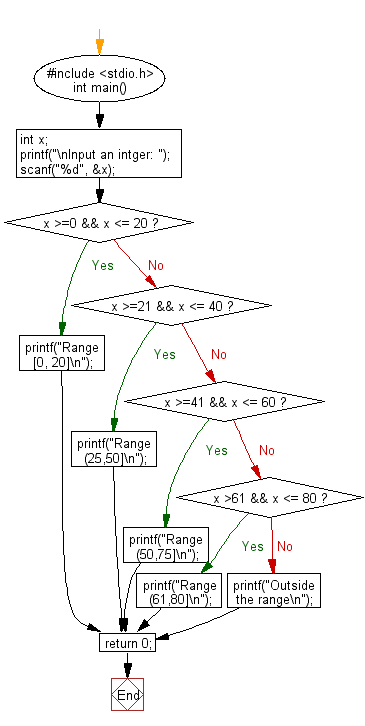﻿ C : Check the specified range where an integer belongs

# C Exercises: Check the specified range where an integer belongs

## C Basic Declarations and Expressions: Exercise-21 with Solution

Write a C program that reads an integer and checks the specified range to which it belongs. Print an error message if the number is negative and greater than 80.
Specified Range: [0, 20], [21, 40], [41, 60], [61, 80]

C Code:

``````#include <stdio.h>
int main() {
int x; // Declare variable to store an integer

// Prompt user for an integer and store in 'x'
printf("\nInput an integer: ");
scanf("%d", &x);

if(x >=0 && x <= 20) // Check if 'x' is in the range [0, 20]
{
printf("Range [0, 20]\n"); // Print message for the first range
}
else if(x >=21 && x <= 40) // Check if 'x' is in the range (21,40]
{
printf("Range (21,40]\n"); // Print message for the second range
}
else if(x >=41 && x <= 60) // Check if 'x' is in the range (41,60]
{
printf("Range (41,60]\n"); // Print message for the third range
}
else if(x >61 && x <= 80) // Check if 'x' is in the range (61,80]
{
printf("Range (61,80]\n"); // Print message for the fourth range
}
else
{
printf("Outside the range\n"); // Print message for values outside of all ranges
}

return 0;
}

``````

Sample Output:

```Input an integer: 15
Range [0, 20]
```

Flowchart:C Programming Code Editor:

What is the difficulty level of this exercise?

Test your Programming skills with w3resource's quiz.

﻿